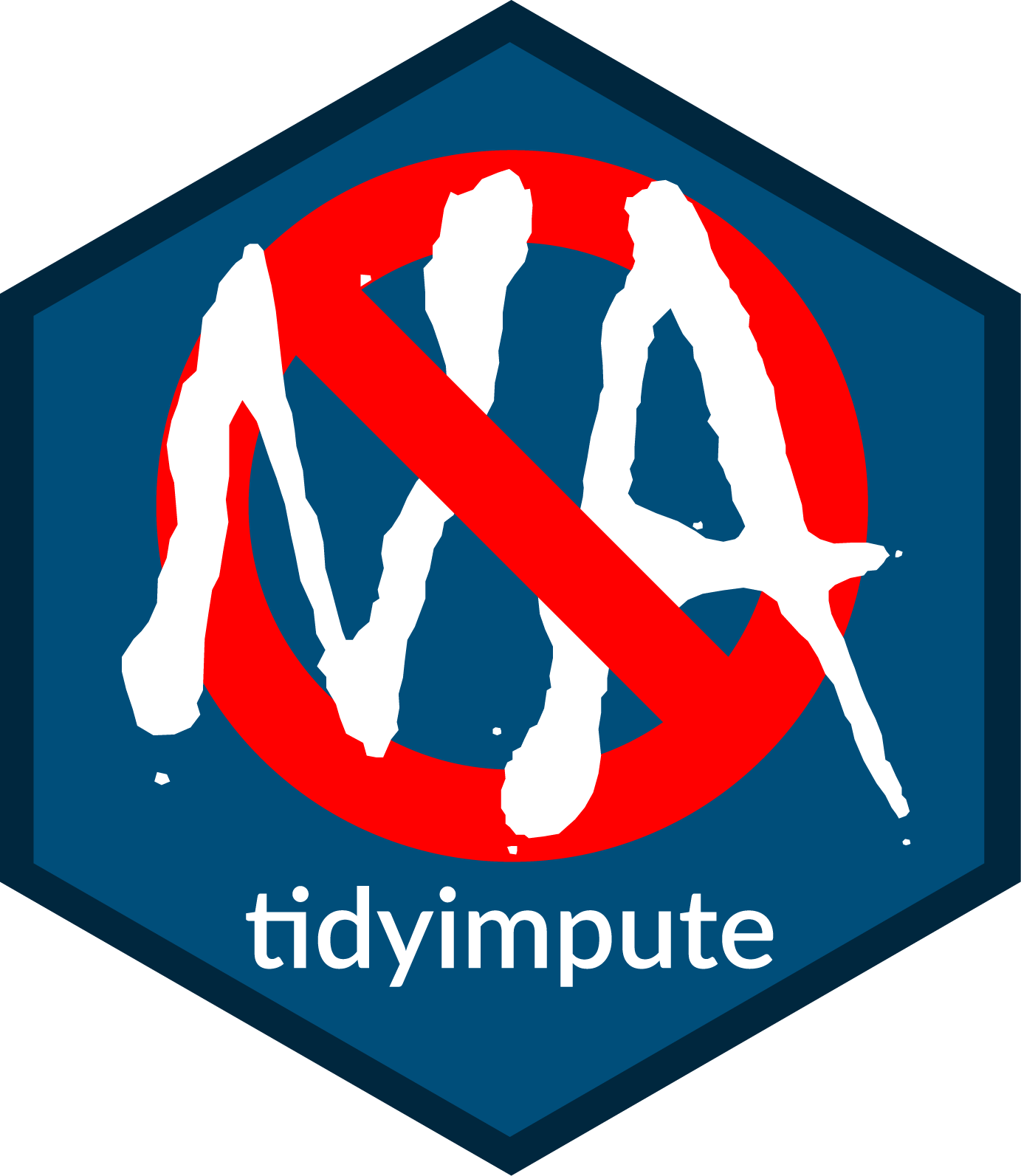## tidyimportComprehensive Library For Handling Missing Values

tidyimpute is tidtverse/dplyr compliant toolkit for imputing missing values (NA) values in list-like and table-like structures including data.tables. It had two goals: 1) extend existing `na.*` functions from the stats packages and 2) provide dplyr/tidyverse compliant methods for tables and lists.

This package is based on the handy na.tools package which provides tools for working with missing values in vectors.

## Feature List

• Over 80 functions for imputi missing values (See [#Function List] below.)
• dplyr/tidyverse compliant inteface:
• `impute_*` family of functions for table- or list-based imputations.
• `impute_*_at`, `impute_*_all` and `impute_*_if` functions
• Uses the **na.tools* package to ensure
• Type/class and length-safe replacement. (tidyimport will never change produce an object with a different length/nrow or type/class of its target.)
• General imputation methods
• Generic imputation: `impute`, `impute_at`, `impute_all`, `impute_if`
• Specialized imputation methods
• Common imputations for:
• constants: `0`, `-Inf`, `Inf`
• univariate, commutative summary functions: `mean`, `median`, `max`, `min`, `zero`
• (Coming Soon) univariate, non-commutive/ordered/time-series data: `loess`, `locf`, `locb`
• (Coming Soon) model-based imputation
• Support for recursive (lists and table-like structures)
• Support for `tibble`
• Support for `data.table`
• Four extensible types of imputations

### Upcoming features

• recall/track which values have been replaced
• `by-group` calculations
• Time-series/ordered/non-commutative methods
• Model-based imputation
• Model-based + by-groups

## Installation

### Github (Development Version)

``````library(devtools)
install_github( "decisionpatterns/tidyimport")``````

### CRAN

``R> install.packages("tidyimpute")``

## Coming Soon …

• Impute by model
• Memorable imputing

## Function List

There are four types of imputation methods. They are distinguished by how the replacement values are calculated. Each is described below as well as describing each of the methods used.

Constants

In “constant” imputation methods, missing values are replaced by an a priori selected constant value. The vector containingmissing values is not used to calculate the replacement value. These take the form: `na.fun(x, ...)`

• `impute_zero` - 0
• `impute_inf` / `impute_neginf` - Inf/-Inf
• `impute_constant` - Impute with a constant

Univariate

(Impute using function(s) of the target variable; When imputing in a table this is also called column-based imputation since the values used to derive the imputed come from the single column alone.)

In “univariate” replacement methods, values are calculated using only the target vector, ie the one containing the missing values. The functions for performing the imputation are nominally univariate summary functions. Generally, the ordering of the vector does not affect imputed values. In general, one value is used to replace all missing values (`NA`) for a variable.

• `impute_max` - maximum
• `impute_minimum` - minumum
• `impute_mean` - mean
• `impute_median` - median value
• `impute_quantile` - quantile value
• `impute_sample` - randomly sampled value via bootstrap.

Ordered Univariate (Coming Soon)

(Impute using function(s) of the target variable. Variable ordering relevant. This is a super class of the previous column-based imputation.)

In “ordered univariate” methods, replacement valuse are calculated from the vector that is assumed to be ordered. These types are very often used with time-series data. (Many of these functions are taken from or patterned after functions in the zoo package.)

• `impute_loess` - loess smoother, assumes values are ordered
• `impute_locf` - last observation carried forward, assumes ordered
• `impute_nocb` - next observation carried backwards, assumes ordered

Multivariate (Coming Soon)

(Impute with multiple variables from the same observation. In tables, this is also called row-based imputation because imputed values derive from other measurement for the same observation. )

In “Multivariate” imputation, any value from the same row (observation) can be used to derive the replacement value. This is generally implemented as a model traing from the data with `var ~ ...`

• `impute_fit`,`impute_predict` - use a model
• `impute_by_group` - use by-group imputation

Generalized (Coming Soon)

(Impute with column and rows.)

Future:

• `unimpute`/`impute_restore` - restore NAs to the vector; remembering replacement
• `impute_toggle` - toggle between `NA` and replacement values

## Examples

``````tbl <- data.frame( col_1 = letters[1:3], col_2=c(1,NA_real_,3), col_3=3:1)

impute( tbl, 2)
impute_mean( tbl )``````